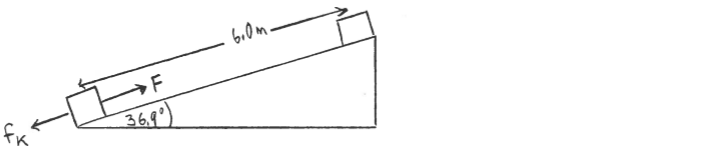# Problem: A box with mass 5.00 kg is pulled up a 36.9° incline by a constant force  F that has magnitude 75.0 N and that is parallel to the incline. The distance along the incline from the bottom to the top is 6.00 m. During the motion of the box, the surface of the incline exerts a constant friction force fk = 18.0 N on the box, in a direction opposite to the motion.For the motion from the bottom of the incline to the top, how much work is done by each of the following forces? In addition to giving the magnitude of the work, be sure to indicate whether the work done is positive or negative.(i) the force F that pulls the box(ii) the friction force fk(iii) the gravity force (iv) the normal force

🤓 Based on our data, we think this question is relevant for Professor Perry's class at TAMU.

###### FREE Expert SolutionView Complete Written Solution
###### Problem Details

A box with mass 5.00 kg is pulled up a 36.9° incline by a constant force  that has magnitude 75.0 N and that is parallel to the incline. The distance along the incline from the bottom to the top is 6.00 m. During the motion of the box, the surface of the incline exerts a constant friction force fk = 18.0 N on the box, in a direction opposite to the motion.

For the motion from the bottom of the incline to the top, how much work is done by each of the following forces? In addition to giving the magnitude of the work, be sure to indicate whether the work done is positive or negative.

(i) the force F that pulls the box

(ii) the friction force fk

(iii) the gravity force

(iv) the normal force